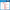Math | Grade 2, 3, 4 | Worksheets

# Two and Three Dimensional Figures Unit Vocabulary Words Interactive Foldables for 3rd Grade – EBOOKAttributes
subject

Grade 2, 3, 4

file

PDF

editable
NoThis set includes 6 vocabulary interactive foldables based on combined state standards for 3rd Grade Two and Three Dimensional Figures Unit. Each set of words is offered in three formats, words only, definitions only, and words with definitions.

These 6 foldables cover:

•Area
•Attributes of three-dimensional figures
•Attributes of two-dimensional figures
•Base of a cone
•Bases of a cylinder
•Bases of a prism
•Classify
•Congruent
•Congruent figures
•Decompose figures
•Edge
•Face of a prism
•Irregular figure
•Polygon
•Properties of three-dimensional figures
•Properties of two-dimensional figures
•Regular figure
•Side
•Sort
•Three-dimensional figure
•Two-dimensional figure
•Unit fraction
•Vertex (vertices) in a three-dimensional figure
•Vertex (vertices) in a two-dimensional figure

This product consists of downloadable a PDF file. Just follow the instructions, cut, fold, glue, and create!

Keywords: math, vocabulary, Area, Attributes of three-dimensional figures, Attributes of two-dimensional figures, Base of a cone, Bases of a cylinder, Bases of a prism, Classify, Congruent, Congruent figures, Decompose figures, Edge, Face of a prism, Irregular figure, Polygon, Properties of three-dimensional figures, Properties of two-dimensional figures, Quadrilateral, Regular figure, Side, Sort, Three-dimensional figure, Two-dimensional figure, Unit fraction, Vertex (vertices) in a three-dimensional figure, Vertex (vertices) in a two-dimensional figure, second grade, third grade, fourth grade, Candie Donner

## What's Included

27 pages in PDF format

## Resource Tags

mathVocabulary WordsAreashapesCongruent figures

### you may also like...

Check out these other great products